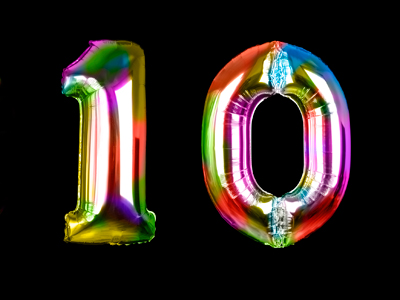In order to change 0.023 to 0.23, we multiply by 10.

# Decimal Numbers (Year 6)

In KS2 Maths, kids learn about decimal numbers. In Year Six, you'll know decimal place value to three spots - tenths, hundredths, and thousandths. Plus, you'll be a pro at adding, subtracting, and rounding decimals. Think metres/cm or £/pence!

Decimals have a point - left side has whole numbers, right side has tenths, hundredths, and thousandths. Decimals are like a cool way to talk about numbers smaller than one. Instead of 1/8, say 0.125: 1 tenth, 2 hundredths, 5 thousandths. Decimals make maths with fractions easier - like metres and kilos, all using tenths, hundredths, and thousandths!

Try a fun quiz to test your decimal number skills!

1.
Which is the decimal fraction equivalent to nine and two thousandths?
9.002
9.2
9.02
0.092
Ones are on the left of the decimal point
2.
What does the digit 1 represent in 0.251?
1 tenth
1 thousandth
1 one
1 hundredth
Remember - tenths, hundredths, thousandths
3.
What is the next number in the sequence 0.007, 0.008, 0.009?
0.001
0.1
0.01
1
The numbers are going up by 0.001, or 11,000 every time
4.
How is 0.7 changed to 0.007?
Multiply by 10
Divide by 100
Divide by 10
Multiply by 100
When we divide by 100 we move the digits two places to the right
5.
What is 1.23m + 63cm?
1.86m
74.23m
7.53m
186m
Convert the cm into metres and you will have 1.23 + 0.63 giving 1.86m
6.
Which is the smallest of these numbers?
0.001
0.1
0.01
0.011
11,000 is less than 111,000, 1100 or 110
7.
What is the figure 8 worth in 2.089?
8 ones
8 hundredths
8 tenths
8 thousandths
2.089 is 2 ones, 0 tenths, 8 hundredths and 9 thousandths
8.
Which is the largest of these numbers?
0.3
0.003
0.033
0.03
310 is larger than 3100, 331,000 or 31,000
9.
Which is a decimal fraction halfway between 6.52 and 6.53?
6.55
6.521
6.525
6.531
There are 10 thousands in a hundredth so half of a hundredth is 5 thousandths
10.
How is 0.023 changed to 0.23?
Multiply by 10
Divide by 100
Divide by 10
Multiply by 100
When we multiply by 10 the digits move one place to the left